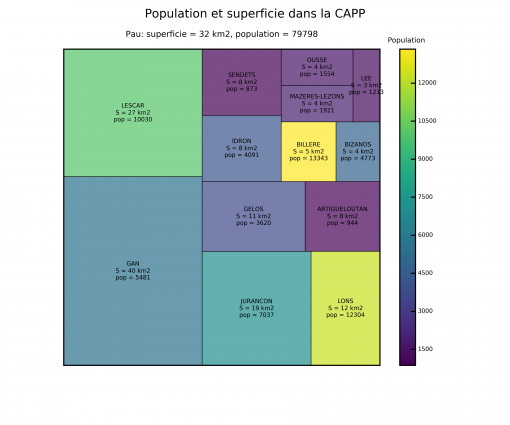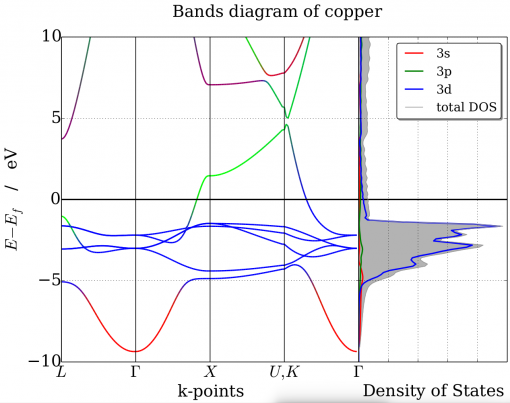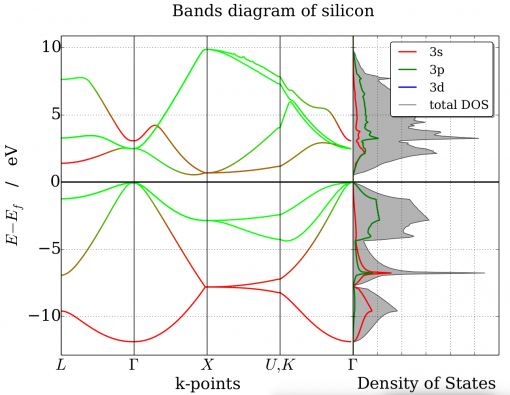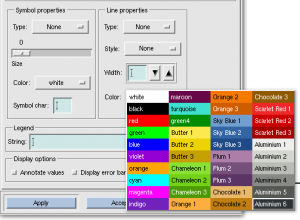## Photoelectric effect gif

Here are provided two simple gif images in order to illustrate the photoelectric effect.

Below the threshold energy, nothing append, whatever the light intensity.

Above the threshold energy, each photon bear enough energy in order to extract electrons from the material.

All pictures can be downloaded here : graphene.zip

## Quickly plot colormap or a color palette with matplotlib

I present here simple convenient functions to plot a matplotlib colormap or a color palette.

The source codes of the functions are available on this gist.

#### For colormaps

You can use either its name or the `matplotlib.cm.colormap` object.

This is how to use the function :

`fig = plot_cmap("summer", 6)`

or

`fig = plot_cmap(plt.cm.summer, 6)`

And you will get :##### The source code
```import matplotlib.pyplot as plt   def plot_cmap(cmap, ncolor): """ A convenient function to plot colors of a matplotlib cmap   Args: ncolor (int): number of color to show cmap: a cmap object or a matplotlib color name """   if isinstance(cmap, str): try: cm = plt.get_cmap(cmap) except ValueError: print("WARNINGS :", cmap, " is not a known colormap") cm = plt.cm.gray else: cm = cmap   with mpl.rc_context(mpl.rcParamsDefault): fig = plt.figure(figsize=(6, 1), frameon=False) ax = fig.add_subplot(111) ax.pcolor(np.linspace(1, ncolor, ncolor).reshape(1, ncolor), cmap=cm) ax.set_title(cm.name) xt = ax.set_xticks([]) yt = ax.set_yticks([]) return fig```

#### For a list of colors

This is how to use the function :

```colors = ["#9b59b6", "#3498db", "#95a5a6", "#e74c3c", "#34495e", "#2ecc71"] show_colors(colors)```

And you will simply get a square for each color :##### The source code
```import matplotlib.pyplot as plt   def show_colors(colors): """ Draw a square for each color contained in the colors list given in argument. """ with plt.rc_context(plt.rcParamsDefault): fig = plt.figure(figsize=(6, 1), frameon=False) ax = fig.add_subplot(111) for x, color in enumerate(colors): ax.add_patch( mpl.patches.Rectangle( (x, 0), 1, 1, facecolor=color ) ) ax.set_xlim((0, len(colors))) ax.set_ylim((0, 1)) ax.set_xticks([]) ax.set_yticks([]) ax.set_aspect("equal")   return fig```

## Treemaps plot using matplotlib and python

Here is an example of a `treemap` plot using `python`, `matplotlib` and the `squarify` module.

The data comes from the open data of the Communauté d’Agglomération Pau-Pyrénées.

The idea is the following :

• The area of each square is the surface area of the towns
• The color scale is over the population in 2011

Gist code here !

#### Output#### Other version

Here is an other version where Pau is present. Nevertheless, I draw a white square background because the population of Pau is huge compared to other towns and thus I did not include it in the color scale.## First principle study of the surface reactivity of layered lithium oxides LiMO2 (M = Ni, Mn, Co)

### Abstract

LiNixMnyCo1−x−yO2 compounds (NMC) are layered oxides widely used in commercial lithium-ion batteries at the positive electrode. Nevertheless surface reactivity of this material is still not well known. As a first step, based on first principle calculations, this study deals with the electronic properties and the surface reactivity of LiMO2 (M = Co, Ni, Mn) compounds, considering the behavior of each transition metal separately in the same α-NaFeO2-type structure, the one of LiCoO2 and NMC. For each compound, after a brief description of the bare slab electronic properties, we explored the acido-basic and redox properties of the (110) and (104) surfaces by considering the adsorption of a gaseous probe. The chemisorption of SO2 produces both sulfite or sulfate species associated respectively to an acido-basic or a reduction process. These processes are localized on the transition metals of the first two layers of the surface. Although sulfate species are globally favored, a different behavior is obtained depending on both the surface and the transition metal considered. We conclude with a simple scheme which describes the reduction processes on the both surfaces in terms of formal oxidation degrees of transition metals.

Vallverdu, G.; Minvielle, M.; Andreu, N.; Gonbeau, D.; Baraille, I. First Principle Study of the Surface Reactivity of Layered Lithium Oxides LiMO2 (M = Ni, Mn, Co). Surface Science 2016, 649, 46–55.

doi:10.1016/j.susc.2016.01.004

## Possible Existence of a Monovalent Coordination for Nitrogen Atoms in LixPOyNz Solid Electrolyte: Modeling of X-ray Photoelectron Spectroscopy and Raman Spectra

### Abstract :

LixPOyNz is an amorphous solid electrolyte widely used in microbattery devices. The present study, based on a confrontation between experiment and theory, aims at providing new knowledge regarding the ionic conductivity model of such systems in correlation with its structure. The computational strategy involved molecular dynamic simulations and first-principle calculations on molecular and periodic models. The experimental target data involve electronic and vibrational properties and were considered through the simulation of Raman and X-ray photoemission spectra in order to identify characteristic patterns of LixPOyNz. In particular, the presence of short phosphate chains is suggested by molecular dynamics calculations, and the simulation of Raman spectra clearly evidenced a new coordination for nitrogen atoms in the amorphous state, not considered until now in the experimental structural model of the electrolyte and initially hypothesized based on core level binding energy computations. Monovalent nitrogen atoms together with short phosphate chains were used to build a structural model of the electrolyte and appeared to lead to a better reproduction of the target experimental results, while it implies a necessary refinement of the diffusion schemes commonly considered for lithium ions.

Guille, É.; Vallverdu, G.; Tison, Y.; Bégué, D.; Baraille, I. Possible Existence of a Monovalent Coordination for Nitrogen Atoms in LixPOyNz Solid Electrolyte: Modeling of X-Ray Photoelectron Spectroscopy and Raman Spectra. J. Phys. Chem. C 2015.

DOI: 10.1021/acs.jpcc.5b08427

## First-principle calculation of core level binding energies of LixPOyNz solid electrolyte

### Abstract :

We present first-principle calculations of core-level binding energies for the study of insulating, bulk phase, compounds, based on the Slater-Janak transition state model. Those calculations were performed in order to find a reliable model of the amorphous LixPOyNz solid electrolyte which is able to reproduce its electronic properties gathered from X-ray photoemission spectroscopy (XPS) experiments. As a starting point, Li2PO2N models were investigated. These models, proposed by Du et al. on the basis of thermodynamics and vibrational properties, were the first structural models of LixPOyNz. Thanks to chemical and structural modifications applied to Li2PO2N structures, which allow to demonstrate the relevance of our computational approach, we raise an issue concerning the possibility of encountering a non-bridging kind of nitrogen atoms (=N-) in LixPOyNz compounds.

Guille, É.; Vallverdu, G.; Baraille, I. First-Principle Calculation of Core Level Binding Energies of LixPOyNz Solid Electrolyte. The Journal of Chemical Physics 2014, 141, 244703.

DOI: 10.1063/1.4904720

## Bands diagram using VASP and pymatgen

This article presents a python source code in order to plot the bands diagram of graphene calculated using VASP. The plot is done using pymatgen library and RGB coloring adapted from this example. Here is the output obtained with the script :

On the band diagram, the contribution of s, (px,py) and pz atomic orbital is map on a RGB scale. Red is associated to a s contribution, green to (px, py) contribution and blue to pz contribution. The same function or procedure can be used to map atomic contributions on the band diagram.

At the end of the article you can find a tarball containing all VASP input/ouput files and the python source code. This calculation is not accurate and is used only for band diagram plotting illustration. For example, you can see a short gap on the DOS which does not exist.

Edit 2016, March 15, update : source code is now python3 and pymatgen version 3.3.5 compatible. You can find the below scripts in an up to date version on github : bandstructureplots

```#!/usr/bin/env python # -*- coding=utf-8 -*-   import sys   import numpy as np from numpy import array as npa   import matplotlib.pyplot as plt from matplotlib.collections import LineCollection from matplotlib.gridspec import GridSpec   import pymatgen as mg from pymatgen.io.vasp.outputs import Vasprun, Procar from pymatgen.symmetry.bandstructure import HighSymmKpath from pymatgen.electronic_structure.core import Spin, Orbital     def rgbline(ax, k, e, red, green, blue, alpha=1.): # creation of segments based on # http://nbviewer.ipython.org/urls/raw.github.com/dpsanders/matplotlib-examples/master/colorline.ipynb pts = np.array([k, e]).T.reshape(-1, 1, 2) seg = np.concatenate([pts[:-1], pts[1:]], axis=1)   nseg = len(k) - 1 r = [0.5 * (red[i] + red[i + 1]) for i in range(nseg)] g = [0.5 * (green[i] + green[i + 1]) for i in range(nseg)] b = [0.5 * (blue[i] + blue[i + 1]) for i in range(nseg)] a = np.ones(nseg, np.float) * alpha lc = LineCollection(seg, colors=list(zip(r, g, b, a)), linewidth=2) ax.add_collection(lc)   if __name__ == "__main__": # read data # ---------   # kpoints labels path = HighSymmKpath(mg.Structure.from_file("./opt/CONTCAR")).kpath["path"] labels = [r"\$%s\$" % lab for lab in path[0:4]]   # bands bands = Vasprun("./bands/vasprun.xml").get_band_structure("./bands/KPOINTS", line_mode=True)   # projected bands data = Procar("./bands/PROCAR").data   # density of state dosrun = Vasprun("./dos/vasprun.xml")   # set up matplotlib plot # ----------------------   # general options for plot font = {'family': 'serif', 'size': 24} plt.rc('font', **font)   # set up 2 graph with aspec ration 2/1 # plot 1: bands diagram # plot 2: Density of State gs = GridSpec(1, 2, width_ratios=[2, 1]) fig = plt.figure(figsize=(11.69, 8.27)) fig.suptitle("Bands diagram of graphene") ax1 = plt.subplot(gs) ax2 = plt.subplot(gs) # , sharey=ax1)   # set ylim for the plot # --------------------- emin = 1e100 emax = -1e100 for spin in bands.bands.keys(): for b in range(bands.nb_bands): emin = min(emin, min(bands.bands[spin][b])) emax = max(emax, max(bands.bands[spin][b]))   emin -= bands.efermi + 1 emax -= bands.efermi - 1 ax1.set_ylim(emin, emax) ax2.set_ylim(emin, emax)   # Band Diagram # ------------   # sum up contribution over carbon atoms data = data[Spin.up].sum(axis=2)   # sum up px and py contributions and normalize contributions contrib = np.zeros((bands.nb_bands, len(bands.kpoints), 3)) for b in range(bands.nb_bands): for k in range(len(bands.kpoints)): sc = data[k][b][Orbital.s.value]**2 pxpyc = data[k][b][Orbital.px.value]**2 + \ data[k][b][Orbital.py.value]**2 pzc = data[k][b][Orbital.pz.value]**2 tot = sc + pxpyc + pzc if tot != 0.0: contrib[b, k, 0] = sc / tot contrib[b, k, 1] = pxpyc / tot contrib[b, k, 2] = pzc / tot   # plot bands using rgb mapping for b in range(bands.nb_bands): rgbline(ax1, range(len(bands.kpoints)), [e - bands.efermi for e in bands.bands[Spin.up][b]], contrib[b, :, 0], contrib[b, :, 1], contrib[b, :, 2])   # style ax1.set_xlabel("k-points") ax1.set_ylabel(r"\$E - E_f\$ / eV") ax1.grid()   # fermi level at 0 ax1.hlines(y=0, xmin=0, xmax=len(bands.kpoints), color="k", lw=2)   # labels nlabs = len(labels) step = len(bands.kpoints) / (nlabs - 1) for i, lab in enumerate(labels): ax1.vlines(i * step, emin, emax, "k") ax1.set_xticks([i * step for i in range(nlabs)]) ax1.set_xticklabels(labels)   ax1.set_xlim(0, len(bands.kpoints))   # Density of state # ----------------   ax2.set_yticklabels([]) ax2.grid() ax2.set_xticks(np.arange(0, 1.5, 0.4)) ax2.set_xticklabels(np.arange(0, 1.5, 0.4)) ax2.set_xlim(1e-6, 1.5) ax2.hlines(y=0, xmin=0, xmax=1.5, color="k", lw=2) ax2.set_xlabel("Density of State")   # s contribution ax2.plot(npa(dosrun.pdos[Orbital.s][Spin.up]) + npa(dosrun.pdos[Orbital.s][Spin.up]), dosrun.tdos.energies - dosrun.efermi, "r-", label="s", linewidth=2)   # px py contribution ax2.plot(npa(dosrun.pdos[Orbital.px][Spin.up]) + npa(dosrun.pdos[Orbital.px][Spin.up]) + npa(dosrun.pdos[Orbital.py][Spin.up]) + npa(dosrun.pdos[Orbital.py][Spin.up]), dosrun.tdos.energies - dosrun.efermi, "g-", label="(px, py)", linewidth=2)   # pz contribution ax2.plot(npa(dosrun.pdos[Orbital.pz][Spin.up]) + npa(dosrun.pdos[Orbital.pz][Spin.up]), dosrun.tdos.energies - dosrun.efermi, "b-", label="pz", linewidth=2)   # total dos ax2.fill_between(dosrun.tdos.densities[Spin.up], 0, dosrun.tdos.energies - dosrun.efermi, color=(0.7, 0.7, 0.7), facecolor=(0.7, 0.7, 0.7))   ax2.plot(dosrun.tdos.densities[Spin.up], dosrun.tdos.energies - dosrun.efermi, color=(0.6, 0.6, 0.6), label="total DOS")   # plot format style # -----------------   ax2.legend(fancybox=True, shadow=True, prop={'size': 18}) plt.subplots_adjust(wspace=0)   # plt.show() plt.savefig(sys.argv.strip(".py") + ".pdf", format="pdf")```

graphene.zip

Hereafter there are two more examples for Cu and Si. The script may be simpler as all data can be extracted using pymatgen methods.

Si_bands.zip

Cu_bands.zip## Configuration de (xm)grace

Grace et plus précisément sa version graphique xmgrace (anciennement xmgr) est un outil permettant de concevoir des graphiques en deux dimensions (XY, polaire, logarithmique, boxplot …) ainsi que faire un peu d’analyse numérique (regression, interpolation, intégration …) et réaliser quelques traitements sur les données. Les graphiques obtenus sont de haute qualité et peuvent être exportés sous divers formats vectoriels (eps, ps, svg) ou non (png, jpg …). Le paquet est disponible sous ubuntu (apt://grace), fedora et la plupart des distributions linux.

site web : plasma-gate.weizmann.ac.il/Grace.

## Accents et séparateur des décimaux

Par défaut, les accents ne sont pas pris en charge. Il faut passer un paramètre de langue au lancement de xmgrace pour pouvoir les utiliser. Sur la ligne de commande, lancer xmgrace de la façon suivante :

`[user@machine] > LANG=C xmgrace`

ou

`[user@machine] > LANG=fr_FR xmgrace`

Suivant votre distribution, le paramètre `LANG=fr_Fr` activera les accents et conservera un séparateur des décimaux avec une virgule, tandis que `LANG=C` activera les accents et changera le séparateur de décimaux en un point. Il est possible d’enregistrer ces paramètres dans un alias pour simplifier l’utilisation. Pour cela, ajouter la ligne suivante dans votre fichier `.bashrc` ou `.profile` :

`alias xm="LANG=fr_FR xmgrace -geometry 1150x900"`

L’option `geometry` permet quand à elle de faire en sorte que la fenêtre de xmgrace soit suffisamment grande pour voir la totalité du graphique.

## Template

Pour éviter d’avoir à saisir systématiquement les mêmes paramètres (épaisseur des bordures, taille du texte …) il est possible de créer un template qui sera chargé automatiquement à l’ouverture du programme. Pour ce faire, créer un graphique vide avec toutes les options qui vous intéressent et sauvegarder le sous le nom `Default.agr`. Placer ensuite ce fichier dans :

`~/.grace/templates/`

Si les dossiers n’existent pas, il faut les créer.

Ce fichier `Default.agr` permet également de définir de nouvelles couleurs pour étoffer un peu la palette proposée par défaut qui est un peu pauvre. Voici, par exemple, les lignes à insérer pour disposer d’une partie des couleurs de bases plus celles de la palette tango. Ces lignes sont à placer à la place de celles définissant les couleurs dans le fichier `Default.agr` (commençant par `@map`. Pour ce faire utiliser un éditeur de texte quelconque tel que vim, gedit, emacs …

```@map color 0 to (255, 255, 255), "white" @map color 1 to ( 0, 0, 0), "black" @map color 2 to (255, 0, 0), "red" @map color 3 to ( 0, 255, 0), "green" @map color 4 to ( 0, 0, 255), "blue" @map color 5 to (148, 0, 211), "violet" @map color 6 to (255, 165, 0), "orange" @map color 7 to ( 0, 255, 255), "cyan" @map color 8 to (255, 0, 255), "magenta" @map color 9 to (114, 33, 188), "indigo" @map color 10 to (103, 7, 72), "maroon" @map color 11 to ( 64, 224, 208), "turquoise" @map color 12 to ( 0, 139, 0), "green4" @map color 13 to (252, 233, 79), "Butter 1" @map color 14 to (237, 212, 0), "Butter 2" @map color 15 to (196, 160, 0), "Butter 3" @map color 16 to (138, 226, 52), "Chameleon 1" @map color 17 to (115, 210, 22), "Chameleon 2" @map color 18 to ( 78, 154, 6), "Chameleon 3" @map color 19 to (252, 175, 62), "Orange 1" @map color 20 to (245, 121, 0), "Orange 2" @map color 21 to (206, 92, 0), "Orange 3" @map color 22 to (114, 159, 207), "Sky Blue 1" @map color 23 to ( 52, 101, 164), "Sky Blue 2" @map color 24 to ( 32, 74, 135), "Sky Blue 3" @map color 25 to (173, 127, 168), "Plum 1" @map color 26 to (117, 80, 123), "Plum 2" @map color 27 to ( 92, 53, 102), "Plum 3" @map color 28 to (233, 185, 110), "Chocolate 1" @map color 29 to (193, 125, 17), "Chocolate 2" @map color 30 to (143, 89, 2), "Chocolate 3" @map color 31 to (239, 41, 41), "Scarlet Red 1" @map color 32 to (204, 0, 0), "Scarlet Red 2" @map color 33 to (164, 0, 0), "Scarlet Red 3" @map color 34 to (238, 238, 236), "Aluminium 1" @map color 35 to (211, 215, 207), "Aluminium 2" @map color 36 to (186, 189, 182), "Aluminium 3" @map color 37 to (136, 138, 133), "Aluminium 4" @map color 38 to ( 85, 87, 83), "Aluminium 5" @map color 39 to ( 46, 52, 54), "Aluminium 6"```

Lorsque vous choisissez une couleur, vous avez maintenant une palette plus importante :## Exportation par défaut

Dans le dossier :

`~/.grace/`

Créer un fichier `gracerc.user` contenant les lignes suivantes pour que le format de sortie par défaut soit `.eps` :

```# HARDCOPY DEVICE "EPS"```

Il sera alors inutile de passer par le menu « print setup » avant d’exporter un graphique. Changer le paramètre `"EPS"` par le format de sortie qui vous convient.

## First-principles calculations of solid-solid interfaces : application to conversion materials for lithium-ion batteries

### Abstract :

Using periodic density functional theory approaches, the thermodynamic stability of solid-solid interfaces generated during the conversion reaction of copper oxide which is a promising electrode material is investigated. Previous experimental results showed that conversion reactions generate a huge proportion of solid-solid interfaces among Cu2O-Cu, Li2O-Cu and Cu2O-Li2O. Interface grand potentials as a function upon the voltage against a Li|Li+ were computed in order to determine the chemical composition of the most stable interfaces. Then a structural model of the electrode material is proposed, based on the work of adhesion of the most stable systems identified in the first step.

L. Martin, G. Vallverdu, H. Martinez, F. Lecras & I. Baraille; First-principles calculations of solid-solid interfaces : application to conversion materials for lithium-ion batteries; Journal of Materials Chemistry, 2012, DOI: 10.1039/C2JM35078E

## Outils pour la symétrie moléculaire : théorie des groupes

En chimie et en physique, on utilise les propriétés de symétrie du système pour simplifier la résolution. L’outils mathématiques à la base de l’utilisation de la symétrie en chimie est la théorie des groupes. Le livre « chimie et théorie des groupes » de Paul H. Walton (édition De Boeck) est un ouvrages bien adapté aux chimistes ou physico-chimistes qui désirent découvrir cette approche.

Lorsqu’on utilise la théorie des groupes, une étape primordiale et très mécanique est la décomposition de la représentation d’un groupe en représentation irréductibles de ce groupe (analogue à déterminer les coordonnées d’un vecteur dans une base orthonormée). Le module python ci-dessous permet d’obtenir automatiquement la décomposition. Voici un exemple :

```>>> from groupes import c4v >>> rep = [4, 0, 0, 2, 0] >>> print(c4v) C4v | 1E 2C4 1C2 2sigma_v 2sigma_d ------------------------------------------------------------ A1 | 1 1 1 1 1 A2 | 1 1 1 -1 -1 B1 | 1 -1 1 1 -1 B2 | 1 -1 1 -1 1 E | 2 0 -2 0 0 >>> c4v.ordre 8 >>> c4v.reduce(rep) 1 A1 + 1 B1 + 1 E```

Je n’ai pas entré tous les groupes ponctuels de symétrie mais uniquement ceux dont j’ai eu besoin pour le moment. Les groupes disponnibles sont :

• C2v
• C3v
• C4v
• D3h
• D4h

groupes.py